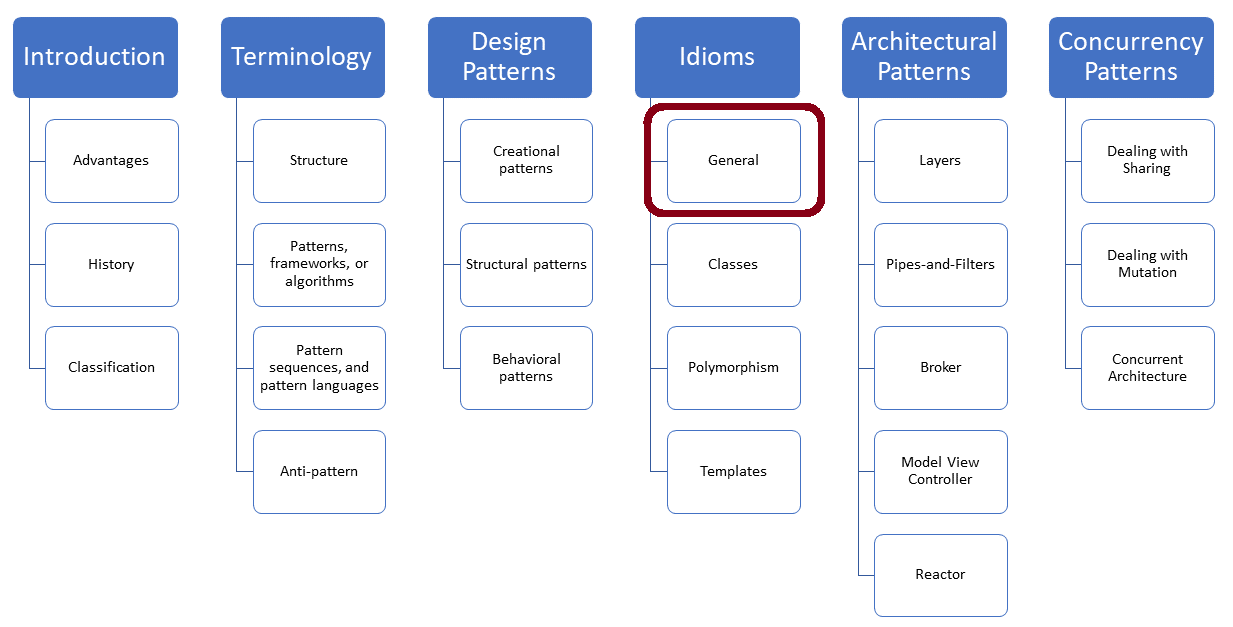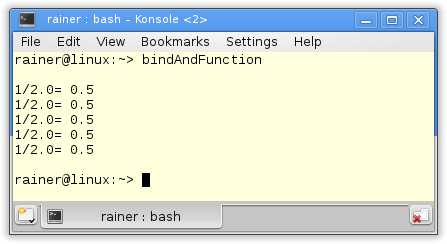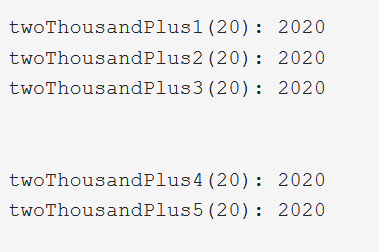# Partial Function Application

Partial Function Application is a technique in which a function binds a few of its arguments and returns a function taking fewer arguments. This technique is related to a technique used in functional languages called currying.A few weeks ago, I had a discussion with a few of my readers. One reader said that I should write about Partial Function Applications. Another reader mentioned that C++ does not support function applications. This is wrong. C++ supports Partial Function Application. Consequently, I am writing today about` std::function`,` std::bind`,` std::bind_front`, lambdas, `auto`, and currying.

## Currying

Partial Function application is quite similar to a technique called currying. Currying is a well-known technique used in functional languages such as Haskell. It stands for a technique in which a function that takes more than one argument will successively be transformed into a series of functions taking only one argument. Therefore, a programming language such as Haskell has only functions taking one argument. I hear your question. How is it possible to implement a function such as `add` which needs two arguments? The magic is happening implicitly. Functions that need n arguments are transformed into functions returning a function that only needs n -1 arguments. The first element is evaluated in this transformation.

The name currying is coined by the mathematician Haskell Curry and Moses Schönfinkel. Currying is named after the family name of Haskell Curry; Haskell after his first name. Sometimes, currying is also called schönfinkeln.

Partial Function Application is more powerful than currying because you can evaluate arbitrary function arguments. Since C++11, C++ supports` std::function` and `std::bind`.

##Modernes C++ Mentoring

Be part of my mentoring programs:

• "Fundamentals for C++ Professionals" (open)
• "Design Patterns and Architectural Patterns with C++" (open)
• "C++20: Get the Details" (reopens December 2023)
• Do you want to stay informed about my mentoring programs: Subscribe via E-Mail.

## `std::bind`

`std::bind` enables you to create callables in various ways. You can

• bind function argument at arbitrary positions,
• reorder the sequence of the function arguments,
• introduce placeholders for function arguments,
• partially evaluate functions.

Furthermore, you can

• directly invoke the new callable,
• use the callable in an algorithm of the Standard Template Library (STL),
• store the callable in `std::function`.

Before I show you an example, I have to introduce `std::function`:

• `std::function` is a polymorphic function wrapper. It can take arbitrary callables and give them a name. Callables are all entities that behave like a function. In particular, these are lambda expressions, function objects, or functions themselves. `std::function` is required if you have to specify the type of a callable.

Now, I’m done with the theory and can show an example of Partial Function Application:

```// bindAndFunction.cpp

#include <functional>
#include <iostream>

double divMe(double a, double b){
return double(a/b);
}

using namespace std::placeholders;                                       // (1)

int main(){

std::cout << '\n';

// invoking the function object directly
std::cout << "1/2.0= " << std::bind(divMe, 1, 2.0)() << '\n';          // (2)

// placeholders for both arguments                                     // (3)
std::function<double(double, double)> myDivBindPlaceholder= std::bind(divMe, _1, _2);
std::cout << "1/2.0= " << myDivBindPlaceholder(1, 2.0) << '\n';

// placeholders for both arguments, swap the arguments                 // (4)
std::function<double(double, double)> myDivBindPlaceholderSwap= std::bind(divMe, _2, _1);
std::cout << "1/2.0= " << myDivBindPlaceholderSwap(2.0, 1) << '\n';

// placeholder for the first argument                                 // (5)
std::function<double(double)> myDivBind1St= std::bind(divMe, _1, 2.0);
std::cout<< "1/2.0= " << myDivBind1St(1) << '\n';

// placeholder for the second argument                                // (6)
std::function<double(double)> myDivBind2Nd= std::bind(divMe, 1.0, _1);
std::cout << "1/2.0= " << myDivBind2Nd(2.0) << '\n';

std::cout << '\n';

}
```

In order to use the simple notation `_1, _2` for the placeholders `std::placeholders::_1, std::placeholders::_2` in the source code, I have to introduce the namespace `std::placeholders` in line 1.

I bind in line 2 in the expression `std::bind(divMe, 1, 2.0)` the arguments` 1` and `2.0` to the function `divMe` and invoke them in place. Lines 3, 4, 5, and 6 follow a similar strategy, but I give the created callables a name using` std::function`, and invoke them finally. A template signature like` double(double, double)` (line 4) or` double(double)` (lines 5 and 6) stands for the type of callable that` std::function` accepts`. double(double, double)` is a function taking two doubles and returning a double.

In particular, the last two examples (lines 5 and 6) in which `std::function` gets a function of arity two and returns a function of arity one is quite astonishing. The arity of a function is the number of arguments a function gets.` std::bind` evaluates in both calls only one argument and uses for the non-evaluated one a placeholder. This technique is called Partial Function Application.

In the end, here is the output of the program.There is another way in C++11 to use Partial Function Application: lambda expressions.

## Lambda Expressions

`std::bind` and `std::function` are almost superfluous with C++11. You can use lambda expressions instead of` std::bind`,  and almost always `auto` instead of `std::function`. Here is the equivalent program based on `auto`, and lambda expressions.

```// lambdaAndAuto.cpp

#include <functional>
#include <iostream>

double divMe(double a, double b){
return double(a/b);
}

using namespace std::placeholders;

int main(){

std::cout << '\n';

// invoking the function object directly
std::cout << "1/2.0= " << [](int a, int b){ return divMe(a, b); }(1, 2.0) << '\n';

// placeholders for both arguments
auto myDivBindPlaceholder= [](int a, int b){ return divMe(a, b); };
std::cout << "1/2.0= " << myDivBindPlaceholder(1, 2.0) << '\n';

// placeholders for both arguments, swap the arguments
auto myDivBindPlaceholderSwap= [](int a, int b){ return divMe(b, a); };
std::cout << "1/2.0= " << myDivBindPlaceholderSwap(2.0, 1) << '\n';

// placeholder for the first argument
auto myDivBind1St= [](int a){ return divMe(a, 2.0); };
std::cout<< "1/2.0= " << myDivBind1St(1) << '\n';

// placeholder for the second argument
auto myDivBind2Nd= [](int b){ return divMe(1, b); };
std::cout << "1/2.0= " << myDivBind2Nd(2.0) << '\n';

std::cout << '\n';

}
```

Let me say write a few words about the lambda expressions. The expression` [](int a, int b){ return divMe(a, b); }(1, 2.0)` defines a lambda exepression that executes `divMe`. The trailing braces invoke the lambda expression just in place using the arguments `1` and `2.0`. On the contrary, the remaining lambda expressions are invoked in the subsequent lines. You can use a lambda expression to bind any argument of the underlying function.

So far, I have applied Partial Function application with `std::bind` and lambda expressions. In C++20, there is a new variation of` std::bind`:

## `std::bind_front`

`std::bind_front `creates a callable`. std::bind_front` can have an arbitrary number of arguments and binds its arguments to the front. You may wonder why we have` std::bind_front` because we have since C++11` std::bind`, which can also bind to the front. Here is the point. First, `std::bind_front` is easier to use because it does not need placeholders, and second,  `std::bind_front` propagates an exception specification of the underlying callable.

The following program exemplifies that you can replace `std::bind_front `with` ``std::bind,` or lambda expressions.

```// bindFront.cpp

#include <functional>
#include <iostream>

int plusFunction(int a, int b) {
return a + b;
}

auto plusLambda = [](int a, int b) {
return a + b;
};

int main() {

std::cout << '\n';

auto twoThousandPlus1 = std::bind_front(plusFunction, 2000);            // (1)
std::cout << "twoThousandPlus1(20): " << twoThousandPlus1(20) << '\n';

auto twoThousandPlus2 = std::bind_front(plusLambda, 2000);              // (2)
std::cout << "twoThousandPlus2(20): " << twoThousandPlus2(20) << '\n';

auto twoThousandPlus3 = std::bind_front(std::plus<int>(), 2000);        // (3)
std::cout << "twoThousandPlus3(20): " << twoThousandPlus3(20) << '\n';

std::cout << "\n\n";

using namespace std::placeholders;

auto twoThousandPlus4 = std::bind(plusFunction, 2000, _1);             // (4)
std::cout << "twoThousandPlus4(20): " << twoThousandPlus4(20) << '\n';

auto twoThousandPlus5 =  [](int b) { return plusLambda(2000, b); };    // (5)
std::cout << "twoThousandPlus5(20): " << twoThousandPlus5(20) << '\n';

std::cout << '\n';

}
```

Each call (lines 1 – 5) gets a callable taking two arguments and returns a callable taking only one argument because the first argument is bound to `2000`. The callable is a function (1), a lambda expression (2), and a predefined function object (line 3). `_1` stands for the missing argument. With lambda expression (line 5), you can directly apply one argument and provide an argument `b` for the missing parameter. Regarding readability, is` std::bind_front` easier to use than `std::bind`, or a lambda expression.## What’s Next?

Argument-Dependent Lookup (ADL), also known as Koening Lookup is a set of “magical” rules for the lookup of unqualified functions based on their function arguments.

Thanks a lot to my Patreon Supporters: Matt Braun, Roman Postanciuc, Tobias Zindl, G Prvulovic, Reinhold Dröge, Abernitzke, Frank Grimm, Sakib, Broeserl, António Pina, Sergey Agafyin, Андрей Бурмистров, Jake, GS, Lawton Shoemake, Jozo Leko, John Breland, Venkat Nandam, Jose Francisco, Douglas Tinkham, Kuchlong Kuchlong, Robert Blanch, Truels Wissneth, Kris Kafka, Mario Luoni, Friedrich Huber, lennonli, Pramod Tikare Muralidhara, Peter Ware, Daniel Hufschläger, Alessandro Pezzato, Bob Perry, Satish Vangipuram, Andi Ireland, Richard Ohnemus, Michael Dunsky, Leo Goodstadt, John Wiederhirn, Yacob Cohen-Arazi, Florian Tischler, Robin Furness, Michael Young, Holger Detering, Bernd Mühlhaus, Matthieu Bolt, Stephen Kelley, Kyle Dean, Tusar Palauri, Dmitry Farberov, Juan Dent, George Liao, Daniel Ceperley, Jon T Hess, Stephen Totten, Wolfgang Fütterer, Matthias Grün, Phillip Diekmann, Ben Atakora, Ann Shatoff, Rob North, and Bhavith C Achar.

Thanks, in particular, to Jon Hess, Lakshman, Christian Wittenhorst, Sherhy Pyton, Dendi Suhubdy, Sudhakar Belagurusamy, Richard Sargeant, Rusty Fleming, John Nebel, Mipko, Alicja Kaminska, Slavko Radman, and David Poole.

 My special thanks to EmbarcaderoMy special thanks to PVS-StudioMy special thanks to Tipi.buildMy special thanks to Take Up Code## Seminars

I’m happy to give online seminars or face-to-face seminars worldwide. Please call me if you have any questions.

### Standard Seminars (English/German)

Here is a compilation of my standard seminars. These seminars are only meant to give you a first orientation.

• C++ – The Core Language
• C++ – The Standard Library
• C++ – Compact
• C++11 and C++14
• Concurrency with Modern C++
• Design Pattern and Architectural Pattern with C++
• Embedded Programming with Modern C++
• Generic Programming (Templates) with C++

#### New

• Clean Code with Modern C++
• C++20

### Contact Me

Modernes C++ Mentoring,Tags:
0 replies